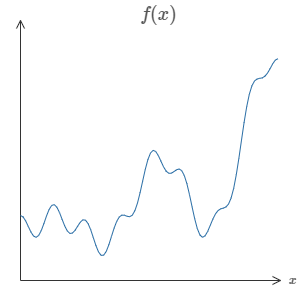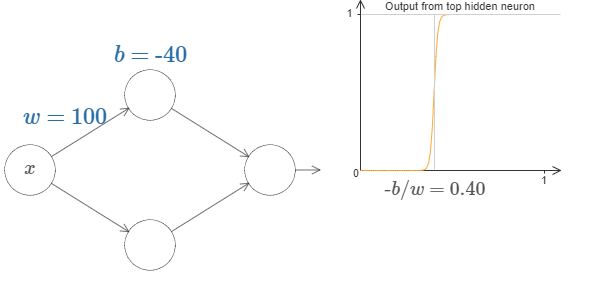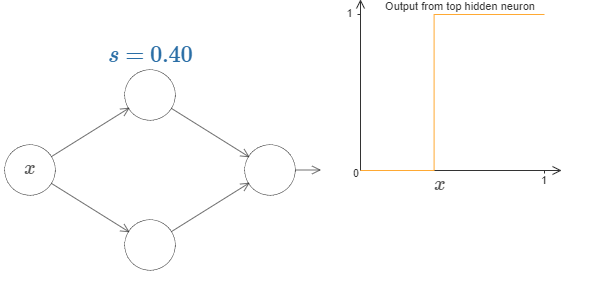# 4.2: Universality with one input and one output

$$\newcommand{\vecs}{\overset { \rightharpoonup} {\mathbf{#1}} }$$ $$\newcommand{\vecd}{\overset{-\!-\!\rightharpoonup}{\vphantom{a}\smash {#1}}}$$$$\newcommand{\id}{\mathrm{id}}$$ $$\newcommand{\Span}{\mathrm{span}}$$ $$\newcommand{\kernel}{\mathrm{null}\,}$$ $$\newcommand{\range}{\mathrm{range}\,}$$ $$\newcommand{\RealPart}{\mathrm{Re}}$$ $$\newcommand{\ImaginaryPart}{\mathrm{Im}}$$ $$\newcommand{\Argument}{\mathrm{Arg}}$$ $$\newcommand{\norm}{\| #1 \|}$$ $$\newcommand{\inner}{\langle #1, #2 \rangle}$$ $$\newcommand{\Span}{\mathrm{span}}$$ $$\newcommand{\id}{\mathrm{id}}$$ $$\newcommand{\Span}{\mathrm{span}}$$ $$\newcommand{\kernel}{\mathrm{null}\,}$$ $$\newcommand{\range}{\mathrm{range}\,}$$ $$\newcommand{\RealPart}{\mathrm{Re}}$$ $$\newcommand{\ImaginaryPart}{\mathrm{Im}}$$ $$\newcommand{\Argument}{\mathrm{Arg}}$$ $$\newcommand{\norm}{\| #1 \|}$$ $$\newcommand{\inner}{\langle #1, #2 \rangle}$$ $$\newcommand{\Span}{\mathrm{span}}$$$$\newcommand{\AA}{\unicode[.8,0]{x212B}}$$

To understand why the universality theorem is true, let's start by understanding how to construct a neural network which approximates a function with just one input and one output:It turns out that this is the core of the problem of universality. Once we've understood this special case it's actually pretty easy to extend to functions with many inputs and many outputs.

To build insight into how to construct a network to compute $$f$$, let's start with a network containing just a single hidden layer, with two hidden neurons, and an output layer containing a single output neuron:To get a feel for how components in the network work, let's focus on the top hidden neuron. In the diagram below, click on the weight, $$w$$, and drag the mouse a little ways to the right to increase $$w$$. You can immediately see how the function computed by the top hidden neuron changes:As we learnt earlier in the book, what's being computed by the hidden neuron is $$σ(wx+b)$$, where $$σ(z)≡1/(1+e−z)$$ is the sigmoid function. Up to now, we've made frequent use of this algebraic form. But for the proof of universality we will obtain more insight by ignoring the algebra entirely, and instead manipulating and observing the shape shown in the graph. This won't just give us a better feel for what's going on, it will also give us a proof*

*Strictly speaking, the visual approach I'm taking isn't what's traditionally thought of as a proof. But I believe the visual approach gives more insight into why the result is true than a traditional proof. And, of course, that kind of insight is the real purpose behind a proof. Occasionally, there will be small gaps in the reasoning I present: places where I make a visual argument that is plausible, but not quite rigorous. If this bothers you, then consider it a challenge to fill in the missing steps. But don't lose sight of the real purpose: to understand why the universality theorem is true. of universality that applies to activation functions other than the sigmoid function.

To get started on this proof, try clicking on the bias, bb, in the diagram above, and dragging to the right to increase it. You'll see that as the bias increases the graph moves to the left, but its shape doesn't change.

Next, click and drag to the left in order to decrease the bias. You'll see that as the bias decreases the graph moves to the right, but, again, its shape doesn't change.

Next, decrease the weight to around $$2$$ or $$3$$. You'll see that as you decrease the weight, the curve broadens out. You might need to change the bias as well, in order to keep the curve in-frame.

Finally, increase the weight up past $$w=100$$. As you do, the curve gets steeper, until eventually it begins to look like a step function. Try to adjust the bias so the step occurs near $$x=0.3$$. The following short clip shows what your result should look like. Click on the play button to play (or replay) the video:

---------------------------------------------------------------------------------------------------VIDEO-----------------------------------------------------------------------------------------------------

We can simplify our analysis quite a bit by increasing the weight so much that the output really is a step function, to a very good approximation. Below I've plotted the output from the top hidden neuron when the weight is $$w=999$$. Note that this plot is static, and you can't change parameters such as the weight.It's actually quite a bit easier to work with step functions than general sigmoid functions. The reason is that in the output layer we add up contributions from all the hidden neurons. It's easy to analyze the sum of a bunch of step functions, but rather more difficult to reason about what happens when you add up a bunch of sigmoid shaped curves. And so it makes things much easier to assume that our hidden neurons are outputting step functions. More concretely, we do this by fixing the weight $$w$$ to be some very large value, and then setting the position of the step by modifying the bias. Of course, treating the output as a step function is an approximation, but it's a very good approximation, and for now we'll treat it as exact. I'll come back later to discuss the impact of deviations from this approximation.

At what value of $$x$$ does the step occur? Put another way, how does the position of the step depend upon the weight and bias?

To answer this question, try modifying the weight and bias in the diagram above (you may need to scroll back a bit). Can you figure out how the position of the step depends on $$w$$ and $$b$$? With a little work you should be able to convince yourself that the position of the step is proportional to $$b$$, and inversely proportional to $$w$$.

In fact, the step is at position $$s=−b/w$$, as you can see by modifying the weight and bias in the following diagram:It will greatly simplify our lives to describe hidden neurons using just a single parameter, $$s$$, which is the step position, $$s=−b/w$$. Try modifying ss in the following diagram, in order to get used to the new parameterization:As noted above, we've implicitly set the weight $$w$$ on the input to be some large value - big enough that the step function is a very good approximation. We can easily convert a neuron parameterized in this way back into the conventional model, by choosing the bias $$b=−ws$$.

Up to now we've been focusing on the output from just the top hidden neuron. Let's take a look at the behavior of the entire network. In particular, we'll suppose the hidden neurons are computing step functions parameterized by step points $$s_1$$ (top neuron) and $$s_2$$ (bottom neuron). And they'll have respective output weights $$w_1$$ and $$w_2$$. Here's the network:What's being plotted on the right is the weighted output $$w_1a_1+w_2a_2$$ from the hidden layer. Here, $$a_1$$ and $$a_2$$ are the outputs from the top and bottom hidden neurons, respectively*

*Note, by the way, that the output from the whole network is $$σ(w_1a_1+w_2a_2+b)$$, where $$b$$ is the bias on the output neuron. Obviously, this isn't the same as the weighted output from the hidden layer, which is what we're plotting here. We're going to focus on the weighted output from the hidden layer right now, and only later will we think about how that relates to the output from the whole network.

These outputs are denoted with as because they're often known as the neurons' activations.

Try increasing and decreasing the step point $$s_1$$ of the top hidden neuron. Get a feel for how this changes the weighted output from the hidden layer. It's particularly worth understanding what happens when $$s_1$$ goes past $$s_2$$. You'll see that the graph changes shape when this happens, since we have moved from a situation where the top hidden neuron is the first to be activated to a situation where the bottom hidden neuron is the first to be activated.

Similarly, try manipulating the step point $$s_2$$ of the bottom hidden neuron, and get a feel for how this changes the combined output from the hidden neurons.

Try increasing and decreasing each of the output weights. Notice how this rescales the contribution from the respective hidden neurons. What happens when one of the weights is zero?

Finally, try setting $$w_1$$ to be $$0.8$$ and $$w_2$$ to be $$−0.8$$. You get a "bump" function, which starts at point $$s_1$$, ends at point $$s_2$$, and has height $$0.8$$. For instance, the weighted output might look like this:Of course, we can rescale the bump to have any height at all. Let's use a single parameter, hh, to denote the height. To reduce clutter I'll also remove the $$"s_1=…"$$ and $$"w_1=…"$$ notations.Try changing the value of $$h$$ up and down, to see how the height of the bump changes. Try changing the height so it's negative, and observe what happens. And try changing the step points to see how that changes the shape of the bump.

You'll notice, by the way, that we're using our neurons in a way that can be thought of not just in graphical terms, but in more conventional programming terms, as a kind of if-then-elsestatement, e.g.:

    if input >= step point:
add 1 to the weighted output
else:
add 0 to the weighted output


For the most part I'm going to stick with the graphical point of view. But in what follows you may sometimes find it helpful to switch points of view, and think about things in terms of if-then-else.

We can use our bump-making trick to get two bumps, by gluing two pairs of hidden neurons together into the same network:I've suppressed the weights here, simply writing the $$h$$ values for each pair of hidden neurons. Try increasing and decreasing both $$h$$ values, and observe how it changes the graph. Move the bumps around by changing the step points.

More generally, we can use this idea to get as many peaks as we want, of any height. In particular, we can divide the interval $$[0,1]$$ up into a large number, $$N$$, of subintervals, and use $$N$$ pairs of hidden neurons to set up peaks of any desired height. Let's see how this works for $$N=5$$. That's quite a few neurons, so I'm going to pack things in a bit. Apologies for the complexity of the diagram: I could hide the complexity by abstracting away further, but I think it's worth putting up with a little complexity, for the sake of getting a more concrete feel for how these networks work.You can see that there are five pairs of hidden neurons. The step points for the respective pairs of neurons are $$0,1/5$$ then $$1/5,2/5$$, and so on, out to $$4/5,5/5$$. These values are fixed - they make it so we get five evenly spaced bumps on the graph.

Each pair of neurons has a value of $$h$$ associated to it. Remember, the connections output from the neurons have weights hh and $$−h$$(not marked). Click on one of the $$h$$ values, and drag the mouse to the right or left to change the value. As you do so, watch the function change. By changing the output weights we're actually designing the function!

Contrariwise, try clicking on the graph, and dragging up or down to change the height of any of the bump functions. As you change the heights, you can see the corresponding change in hh values. And, although it's not shown, there is also a change in the corresponding output weights, which are $$+h$$ and $$−h$$.

In other words, we can directly manipulate the function appearing in the graph on the right, and see that reflected in the $$h$$ values on the left. A fun thing to do is to hold the mouse button down and drag the mouse from one side of the graph to the other. As you do this you draw out a function, and get to watch the parameters in the neural network adapt.

Time for a challenge.

Let's think back to the function I plotted at the beginning of the chapter:I didn't say it at the time, but what I plotted is actually the function

$f(x)=0.2+0.4x^2+0.3xsin(15x)+0.05cos(50x),\label{113}\tag{113}$

plotted over $$x$$ from $$0$$ to $$1$$, and with the $$y$$ axis taking values from $$0$$ to $$1$$.

That's obviously not a trivial function.

You're going to figure out how to compute it using a neural network.

In our networks above we've been analyzing the weighted combination $$\sum_j{w_ja_j}$$ output from the hidden neurons. We now know how to get a lot of control over this quantity. But, as I noted earlier, this quantity is not what's output from the network. What's output from the network is $$σ(\sum_j{w_ja_j+b})$$ where $$b$$ is the bias on the output neuron. Is there some way we can achieve control over the actual output from the network?

The solution is to design a neural network whose hidden layer has a weighted output given by $$σ^{−1}∘f(x)$$, where $$σ^{−1}$$ is just the inverse of the $$σ$$ function. That is, we want the weighted output from the hidden layer to be:If we can do this, then the output from the network as a whole will be a good approximation to $$f(x)$$*

*Note that I have set the bias on the output neuron to $$0$$.

Your challenge, then, is to design a neural network to approximate the goal function shown just above. To learn as much as possible, I want you to solve the problem twice. The first time, please click on the graph, directly adjusting the heights of the different bump functions. You should find it fairly easy to get a good match to the goal function. How well you're doing is measured by the average deviation between the goal function and the function the network is actually computing. Your challenge is to drive the average deviation as low as possible. You complete the challenge when you drive the average deviation to $$0.40$$ or below.

Once you've done that, click on "Reset" to randomly re-initialize the bumps. The second time you solve the problem, resist the urge to click on the graph. Instead, modify the $$h$$ values on the left-hand side, and again attempt to drive the average deviation to $$0.40$$ or below.You've now figured out all the elements necessary for the network to approximately compute the function $$f(x)$$! It's only a coarse approximation, but we could easily do much better, merely by increasing the number of pairs of hidden neurons, allowing more bumps.

In particular, it's easy to convert all the data we have found back into the standard parameterization used for neural networks. Let me just recap quickly how that works.

The first layer of weights all have some large, constant value, say $$w=1000$$.

The biases on the hidden neurons are just $$b=−ws$$. So, for instance, for the second hidden neuron $$s=0.2$$ becomes $$b=−1000×0.2=−200$$.

The final layer of weights are determined by the $$h$$ values. So, for instance, the value you've chosen above for the first $$h$$, $$h= -0.3$$, means that the output weights from the top two hidden neurons are $$-0.3$$ and $$0.$$, respectively. And so on, for the entire layer of output weights.

Finally, the bias on the output neuron is $$0$$.

That's everything: we now have a complete description of a neural network which does a pretty good job computing our original goal function. And we understand how to improve the quality of the approximation by improving the number of hidden neurons.

What's more, there was nothing special about our original goal function, $$f(x)=0.2+0.4x^2+0.3sin(15x)+0.05cos(50x)$$. We could have used this procedure for any continuous function from $$[0,1]$$ to $$[0,1]$$. In essence, we're using our single-layer neural networks to build a lookup table for the function. And we'll be able to build on this idea to provide a general proof of universality.

This page titled 4.2: Universality with one input and one output is shared under a CC BY-NC 3.0 license and was authored, remixed, and/or curated by Michael Nielson via source content that was edited to the style and standards of the LibreTexts platform; a detailed edit history is available upon request.